# 30+ Subtraction Printables & Activities

There are so man words that mean subtraction for kids to learn. And there are so many ways to teach subtraction. I thought I would round up all my fun subtraction printables and activities on the site.

I love having a mix of things you can use and I love having them all in one place that is easy to find.Subtraction goes hand in hand with addition. A lot of the printables have a mix of addition and subtraction. But there are some printables just subtraction.

## Things to use with the Subtraction Printables

There are some of the most commonly used items with these printables. I always get asked what I use and it is this list.

• printer• copy paper• white cardstock• color cardstock• laminating pouches• laminator• crayons• pencil• unifix cubes• clear spinners• clothespins• 1 inch ring• 6 sided double dice• 12-sided double diceYou are going to find no-prep printables, hands-on learning, help mats, flashcards, and more in the set. I have divided them up below in different sections to help you find what you need.

## Subtraction Cards & Bookmarks

These are the basic hands-on flashcards and bookmarks that you can use with subtraction. They are simple and easy to print and use.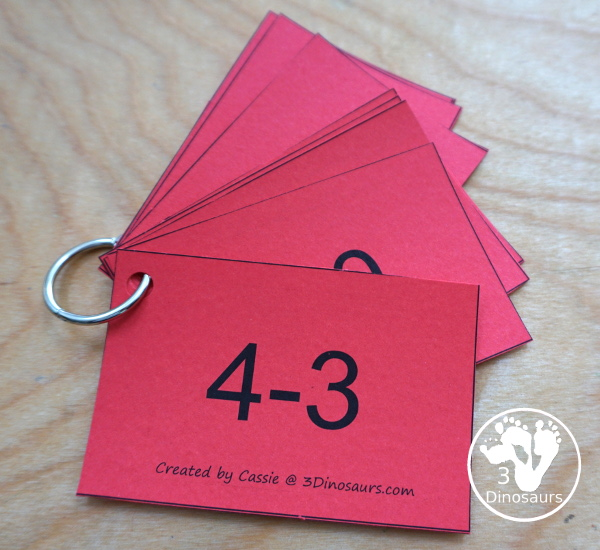Subtraction Flash Cards 1 to 10 have all the ways to subject from that number. It has five colors to pick from.

Subtraction Flash Cards 11 to 20 have like the 1 to 10 all the ways to subject from that number from 11 to 20 in the same five color options.

Subtraction Bookmarks has subtraction from each number from 1 to 20 with all the options on one bookmark.

## Subtraction Sorting, Clip Cards & Task Cards

These are all mix of different types of subtraction cards you can use with kids. These are great math centers for subtraction.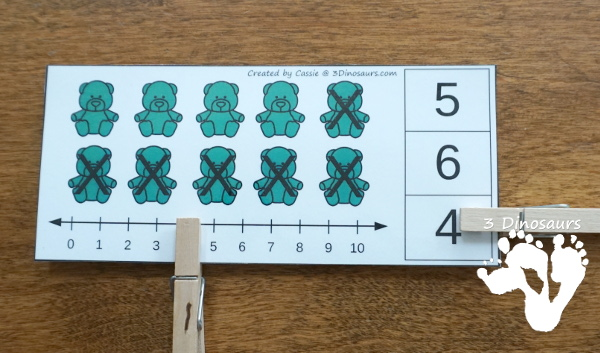Crayon Themed Subtraction Sorting has sorting the crayon to boxes with the correct number.

Bear Themed Addition, & Subtraction Clip Cards are great clip cards with a numberline to help with the subtraction!

Flower Subtraction Task Cards has 12 cards for working on subtraction. It has two type of cards and a recording sheet for the 12 cards.

Garden Themed Subtraction Cards has pictures with space to write the equation below.

Winter Subtraction Clip Cards are one of my favorite. I think I just love clip cards!

Ice Cream Cone Addition & Subtraction Fact Family Matching works on addition and subtraction from 1 to 20 with matching ice cream cones and scoops.

Space Themed Subtraction Puzzle has subtraction from 1 to 20 with a fun puzzles for kids to solve.

## Subtraction Mats & Helps

A fun collection of mats and helps for subtraction. A lot of these match up with addition versions as well. You can see each of the types below. You have charts, math fact houses, subtracting mats and more all great ways to work on subtraction.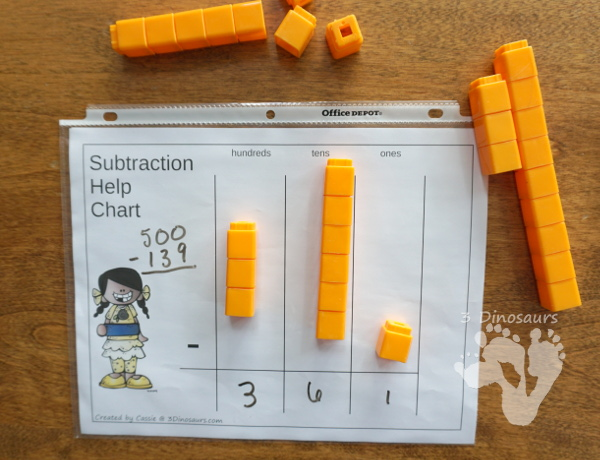Math Fact House: Addition & Subtraction has several color options for kids to work on their mat facts.

Using Place Value Mats for Addition & Subtraction can show you how to use place value for subtracting numbers.

Addition & Subtraction Number Bond Mats with 100 Chart makes it easy to do subtraction with bigger numbers when you use the chart.

Hands on Number Bond Mats: Addition & Subtraction is a great beginners mat for working on subtraction

Math Number Bond Mats: Addition & Subtraction are fun number bond mats with number lines to help with subtraction.

### Seasonals Subtraction Mats

These fun seasonal subtraction mats are great for working with subtracting from 20. You have a spinner and a ten frame mat to work with. Each has a great theme for kids to use.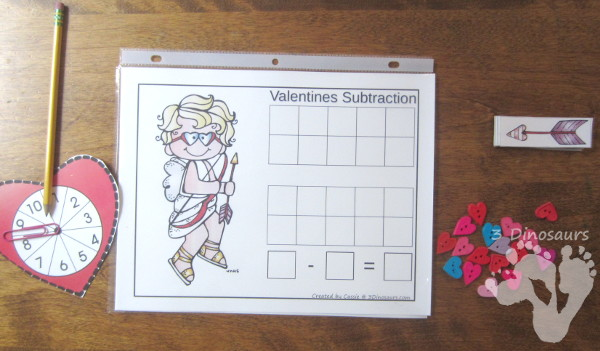Hands-On Beach Themed Addition & Subtraction Mats

Valentines Themed Addition & Subtraction Mats

Christmas Themed Addition & Subtraction Mats

Thanksgiving Math With Addition & Subtraction

Dinosaur Themed Addition & Subtraction Mats

Apple Themed Addition & Subtraction Mats

## Subtraction Printable Worksheets

Spinning Subtraction: Ways to Subtract 1 to 10 This has each number and you can practice subtracting from that number.

Christmas Themed Word Printables has some subtraction wordproblems for kids to work on.

### Seasonal Subtraction No-Prep Worksheets

There are some seasonal no-prep worksheets that have 30 days worth of daily math of addition and subtraction. They are great for morning work, homework or those that need just a little extra practice.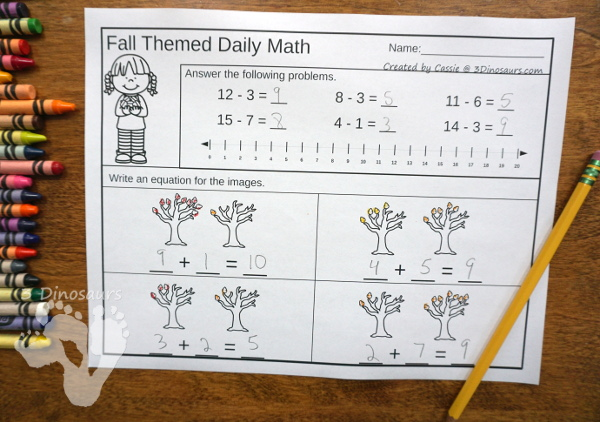Ocean No-Prep Math: Addition & Subtraction

Spring Themed No-Prep Math: Addition & Subtraction

St Patrick’s Day No-Prep Math: Addition & Subtraction

No-Prep Valentines Math Printables For Addition & Subtraction

No-Prep Winter Printables: Addition & Subtraction

Thanksgiving No-Prep Math Packs: Addition & Subtraction

No-Prep Candy & Halloween Math Packs: Addition & Subtraction

No-Prep Math Packs For Fall: Fall, Apples and Pumpkins: Addition & Subtraction

School Themed No-Prep Math

Zoo Themed No-Prep Math Printables

## Check out these other types of Math:

Multiplication Printable & Activities

Division Printables & Activities

Skip Counting Printables & Activities

Cassie – 3Dinosaurs.com